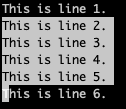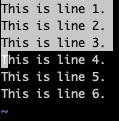## 1. Overview

Joining lines without spaces between them in Vim is crucial to solving use cases such as merging paragraphs, concatenating strings, etc.

In this tutorial, we’ll learn multiple ways to join lines in a text file without introducing a space.

## 2. Scenario Setup

Let’s start by looking at the data.txt sample file containing multiple lines of text:

``````\$ cat data.txt
This is line 1.
This is line 2.
This is line 3.
This is line 4.
This is line 5.
This is line 6.``````

In the following sections, we’ll learn how to join lines in data.txt with examples, each using the original file content. All the approaches we’ll see preserve any spaces that are already present at the start or end of the line(s).

## 3. Using the join Command

In this section, we’ll learn how to solve our use case of joining lines without introducing a space with the help of the join command.

### 3.1. Basics

First, let’s start by looking at the syntax for the join command:

``````:[line number]j[oin][!] [line count]
or
:[line range]j[oin][!]
``````

We can notice a colon (:) at the start of the command usage, so we can only use the join command in the command-line (ex) mode.

By default, the join command adds space between the lines. To join lines without adding a space, we can use the ! flag. Furthermore, we can specify a line count to join multiple lines.

### 3.2. Joining Current Line With Subsequent Lines

On opening the data.txt file in the Vim editor, by default, our current line will be the first line of the file. So, let’s see the join command in action:

``:join``

On execution of the command, we can see that the first and second lines are joined with spaces between them:

``````This is line 1.  This is line 2.
This is line 3.
This is line 4.
This is line 5.
This is line 6.``````

We can also see that if the line ends with a period (.), then two spaces are introduced. Otherwise, the default behavior is to introduce a single space.

Now, let’s execute join! to avoid the addition of spaces as line separators:

``:join!``

We can see that this time, lines are joined without extra space between them:

``````This is line 1.This is line 2.
This is line 3.
This is line 4.
This is line 5.
This is line 6.``````

Lastly, let’s specify 2 as the line number and 3 as the line count with the join! command:

``:2join! 3``

On execution of the command, we can see that starting from line number 2, three lines are joined together without any spaces:

``````This is line 1.
This is line 2.This is line 3.This is line 4.
This is line 5.
This is line 6.``````

Great! It looks like we’ve got this one right.

### 3.3. Joining Range of Lines

Now, let’s specify a range of lines with the join! command:

``:3,4join!``

After execution of the command, we’ll find that all lines matching the range are joined together without any spaces:

``````This is line 1.
This is line 2.
This is line 3.This is line 4.
This is line 5.
This is line 6.``````

Perfect! We’ve got the expected result where lines in the [3,4] range are joined together.

### 3.4. Joining a Selection of Lines

We can also apply the join command to a selection of lines. For this purpose, let’s imagine that we’ve selected lines [2,5] in the visual mode using either the v or gh keys, together with the down arrow key:Now, when we go into the ex-mode by pressing the : key, we’ll see that a line range is pre-populated:

``:'<,'>``

We must note that ‘<,’> represents the selection of lines. So, let’s go ahead and execute join! for this range:

``:'<,'>join!``

As expected, we can see that the selected lines in the range [2,5] are joined together with the immediate line  without any spaces:

``````This is line 1.
This is line 2.This is line 3.This is line 4.This is line 5.This is line 6.``````

## 4. Using the gJ Command

In this section, let’s learn how to join lines without introducing any space using the gJ command in Vim’s normal mode.

### 4.1. J and gJ Commands

Let’s start by seeing the usage for the J command that we can use to join lines in Vim’s normal mode:

``[count][g]J``

Although the J command joins lines with a space by default, we can modify it with the g modifier to join lines without spaces. We can also choose how many lines to join together.

Now, let’s say we’re on the first line currently. On pressing the J key, we’ll see that the first and second lines join while introducing spaces between them:

``````This is line 1.  This is line 2.
This is line 3.
This is line 4.
This is line 5.
This is line 6.``````

On the other hand, if we press the gJ keys together, the lines join without introducing any spaces between them:

``````This is line 1.This is line 2.
This is line 3.
This is line 4.
This is line 5.
This is line 6.``````

So, let’s remember that g modifies the J command to avoid introducing any spaces on joining lines.

Lastly, let’s use the 3gJ command in the normal mode to see that a total of three lines starting from the current line are joined together:

``````This is line 1.This is line 2.This is line 3.
This is line 4.
This is line 5.
This is line 6.``````

### 4.2. Joining a Selection of Lines

We can also use the gJ command on a selection of lines. To demonstrate this, let’s select the first three lines of the file using either the v key, together with the down arrow key:Now, on pressing the gJ keys, we’ll see that the selected lines will join together with the immediate next line:

``````This is line 1.This is line 2.This is line 3.This is line 4.
This is line 5.
This is line 6.``````

Fantastic! It looks like we’ve nailed this one!

## 5. Using the s Command

We can also use the s command to join multiple lines together without introducing any spaces between them:

``:[line range]s/\$\n//``

We must note that the command joins the lines in the specified range by replacing line breaks with empty characters.

First, let’s start by executing the command without explicit mention of a line range:

``:s/\$\n//``

On successful execution of the command, we can see that the first two lines are joined together because the specified range only includes the current line:

``````This is line 1.This is line 2.
This is line 3.
This is line 4.
This is line 5.
This is line 6.``````

Next, let’s specify [2,3] as the range of lines for the substitution:

``:2,3s/\$\n//``

As expected, we’ll find that lines in the [2,3] range are joined together with the 4th line in the file:

``````This is line 1.
This is line 2.This is line 3.This is line 4.
This is line 5.
This is line 6.``````

Lastly, let’s look at a special scenario where we use the % character to specify the range of lines:

``:%s/\$\n//``

It’s interesting to note that all the lines are joined together because % represents the entire range of lines in the current file:

``This is line 1.This is line 2.This is line 3.This is line 4.This is line 5.This is line 6.``

## 6. Using a Macro

In vi, a macro is a recorded sequence of commands that we can replay on demand. In this section, let’s learn how to record and replay the macro for joining lines.

### 6.1. Recording the Macro

First, we must ensure that we’re in the normal mode by pressing the Esc key. Then, we need to press the qj keys together to start recording the macro in the j register:

``qj``

We must note that we can use any of the named registers [a-zA-Z] we aren’t using for any other purpose.

Now, we’ll be able to verify the status in the lower left column of the window:

``recording @j``

Next, let’s press the gJ keys together to join the current line with the immediate next line:

``````This is line 1.This is line 2.
This is line 3.
This is line 4.
This is line 5.
This is line 6.``````

As expected, the first and second lines are joined without spaces between them. Additionally, these commands in the same sequence are being recorded.

Finally, let’s stop the macro recording by pressing the q key.

### 6.2. Replaying the Macro

Whenever we want to replay the macro, we must ensure that we’re in vi’s normal mode by pressing the Esc key. After that, we can press the @j keys together to replay the macro from the j register.

Let’s see this in action while we’re on the first line of the file:

``````This is line 1.This is line 2.This is line 3.
This is line 4.
This is line 5.
This is line 6.``````

As we’re replaying the macro after joining the first two lines, we can see that the original third line is joined together.

Further, let’s go to the second line in the file and execute the @j macro with a count of 2:

``2@j``

We can see that the macro is replayed twice, joining the immediate two lines from the current line:

``````This is line 1.This is line 2.This is line 3.
This is line 4.This is line 5.This is line 6.``````

That’s it! We’ve successfully used macros to solve our use case in hand.

## 7. Conclusion

In this article, we learned how to join multiple lines in Vim without adding spaces using commands like join, gJ, and s. Additionally, we solved the use case with macros.